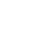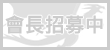LV. 34
GP 357# 《林詩的心願》(２)　遇見~暗戀的心

「喔！真是對不起，魚魚喵！」林詩還真的很認真地跟那隻餐桌上的魚道歉。

「討厭喵！妳欺負我喵！」林詩小生氣地說。

「對…對不起，不過妳到底在想什麼？飯也沒好好吃。」坦絲修女收回笑容，關心地問。

「……」林詩不知該不該說。

「若是真的不想講，妳可以不用講的。」坦絲修女勸道。

「……對不起喵。」林詩實在不想讓坦絲修女擔憂她的事情。

「沒關係，反而是我該跟妳說對不起呢！」坦絲修女笑笑，心想自己總有一天能夠讓林詩不再為她自己的外貌而感到自卑。

「啊！真是糟糕！我竟然也忘了……」今天是林詩在皇立聖斯蒙料理學院第二學年的開學日，坦絲修女因為牽掛林詩的心事而忘了這件事情。

「東西都帶齊了嗎？」坦絲修女問。

「應該吧喵！我要出門了喵！」林詩雖然不確定東西是否帶齊了，但是她要遲到也顧不了那麼多了。

「嗯…小心點喔！」坦絲修女關心地說。

=^.^=~=^.^=~=^.^=~=^.^=~=^.^=~=^.^=~=^.^=~=^.^=~=^.^=~=^.^=~=^.^=~=^.^=

「喔！」「唉唷喵！」兩人同時發出聲音。

「對不起…對不起…妳沒事吧…林詩？！」對方先從地上站起，想要攙扶被他撞倒的人時，卻發覺原來是自己認識的人。

「怎麼了？林詩，妳還好吧？」拉杜看她老半天沒回神，他還以為自己把對方給撞傻了。

「嗯…好喵…」林詩微紅著臉，輕輕地答著。

(To be continuated……)

=^.^=~=^.^=~=^.^=~=^.^=~=^.^=~=^.^=~=^.^=~=^.^=~=^.^=~=^.^=~=^.^=~=^.^=

To唯弟：face基於日前微軟官方表示 Internet Explorer 不再支援新的網路標準，可能無法使用新的應用程式來呈現網站內容，在瀏覽器支援度及網站安全性的雙重考量下，為了讓巴友們有更好的使用體驗，巴哈姆特即將於 2019年9月2日 停止支援 Internet Explorer 瀏覽器的頁面呈現和功能。

。Google Chrome（推薦）
。Mozilla Firefox
。Microsoft Edge（Windows10以上的作業系統版本才可使用）

face我們了解您不想看到廣告的心情⋯ 若您願意支持巴哈姆特永續經營，請將 gamer.com.tw 加入廣告阻擋工具的白名單中，謝謝 !【教學】